Please review your test results by moving cursor to answer sheet icon.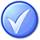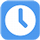1.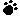What is the difference between a histogram and a pareto chart?

A) A histogram is a less accurate version of a pareto chart.
B) A histogram is a type of pareto chart.
C) A pareto chart is a type of histogram.
D) A histogram and a pareto chart simply different terms for the same technique.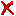Your answer=D, Correct answer=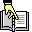, Work Sheet:

2.A)
B)
C)
D)

Your answer=, Correct answer=,

3.A)
B)
C)
D)

Your answer=, Correct answer=,

4.Which of these is not a tool or technique for the Administer Procurements process?

A) Contract change control system
B) Recommended Corrective ActionYour answer=D, Correct answer=, Work Sheet:
5.Your answer=, Correct answer=,